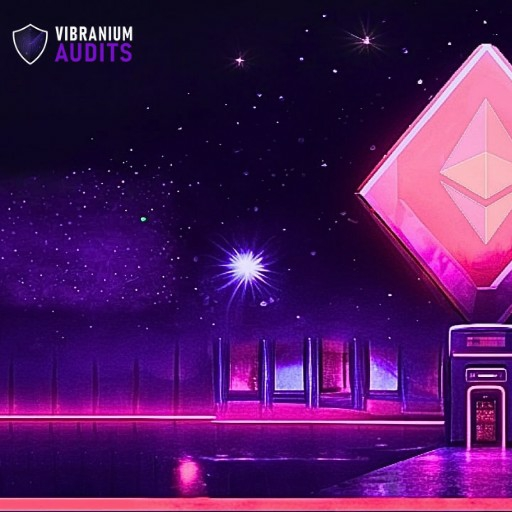# Gas Optimization in Ethereum Smart Contracts: 10 Best Practices

Introduction

Gas optimization is a critical aspect of Ethereum smart contract development to enhance efficiency, reduce costs, and ensure scalability. This article presents ten best practices with diagrams, formulas, and code examples to achieve optimal gas usage in Ethereum smart contracts.

1. Minimize Complex Computations

Complex computations consume more gas. Optimize algorithms and avoid nested loops to reduce gas costs.

2. Use uint instead of int

Unsigned integers (uint) consume less gas than signed integers (int).

3. Limit External Calls

Minimize external calls to other contracts to reduce gas consumption.

4. Use Memory Efficiently

Use memory (memory variables) instead of storage (state variables) for temporary data to save gas.

5. Apply Looping Carefully

Optimize loops to reduce gas costs. Use fixed-size loops when possible.

6. Implement Lazy Evaluation

Lazy evaluation can save gas by deferring computations until necessary.

7. Optimize Data Structures

Choose gas-efficient data structures. Mappings are more efficient for key-value storage.

8. Use Libraries

Reuse external libraries to avoid redundant code and reduce gas consumption.

9. Avoid String Operations

String operations are gas-expensive. Minimize their use and prefer numerical data types.

10. Test and Monitor Gas Usage

Regularly test on test networks and monitor gas consumption using tools like GasGuzzler.

Formulas

1. Gas Cost Formula:

Gas Cost = Base Gas Cost + (Gas Cost per Byte * Transaction Data Size) + (Gas Cost for Opcode * Number of Instructions)

Code Example

Let's implement a simple smart contract to illustrate some of the gas optimization best practices:

pragma solidity ^0.8.0;

contract GasOptimization {

uint256 public totalValue;

function calculateSum(uint256[] memory numbers) public {

uint256 sum = 0;

for (uint256 i = 0; i < numbers.length; i++) {

sum += numbers[i];

}

totalValue = sum;

}

}

In this contract, we have a function calculateSum that calculates the sum of an array of numbers. Let's analyze the gas optimization best practices in this code:

1. Minimize Complex Computations: The function uses a simple loop to calculate the sum, avoiding complex computations.
2. Use uint instead of int: The totalValue variable is declared as a uint256, saving gas compared to using a signed integer.
3. Use Memory Efficiently: The numbers array is passed as a memory variable, reducing gas usage compared to using storage.
4. Apply Looping Carefully: The loop iterates through the array of numbers, but since the loop is not excessively large, it won't cause high gas consumption.

Conclusion

By following these best practices and understanding gas cost formulas, developers can optimize their Ethereum smart contracts for efficient execution. Efficient gas usage enhances the scalability and cost-effectiveness of DApps, contributing to a better user experience and broader adoption of blockchain technology.